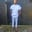Related Tags

number method
clojure
community creator
communitycreator

# How to use the number method in Clojure numbersChinweuba Elijah Azubuike

### What is the number method?

The number method is a number test method. It is used to test if a number is a number and not a string or any other data type.

### Syntax

(number? number)
Syntax for number method

### Parameter

The number method accepts just one parameter, which it will test to see if it is a number.

### Return value

The number method returns true if the value passed is a number. It will return false if the value is not a number.

### Usage

We use this method to avoid unnecessary errors arising while carrying out calculations involving numbers.

### Code

(ns clojure.examples.hello
(:gen-class))

(defn numba []
(def x (number? :a))
(println x)

(def x (number? -1))
(println x)

(def x (number? 9))
(println x))
(numba)
An example of the number method

### Explanation

• Line 5: We define a function numba.
• Line 6: We pass a into the number method.
• Line 7: We print the output and notice that the output we get is false because a is not a number.
• Line 9: We pass -1 into the number method.
• Line 10: We print the output and notice that the output we get is true because -1 is a number even though it is negative.
• Line 12: We pass 9 into the number method.
• Line 13: We print the output and notice that the output we get is true because 9 is a number.
• Line 14: We call our numba function to execute the code.

RELATED TAGS

number method
clojure
community creator
communitycreator

CONTRIBUTORChinweuba Elijah Azubuike
RELATED COURSES

View all Courses

Keep Exploring

Learn in-demand tech skills in half the time